TitleCollege Algebra
Answer/Discussion to Practice Problems
Tutorial 7: Factoring PolynomialsAnswer/Discussion to 1a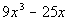The first thing that we always check when we are factoring is WHAT? The GCF.  In this case, there is one.  Factoring out the GCF of x we get: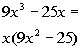*Factor an x out of every term

 Next, we assess to see if there is anything else that we can factor. This fits the form of the difference of two squares.  So we will factor using that rule: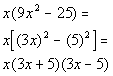*Fits the form of a diff. of two squares *Factor as the prod. of sum and diff. of bases

 Note that if we would multiply this out and the original expression out we would get the same polynomial.Answer/Discussion to 1b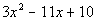The first thing that we always check when we are factoring is WHAT? The GCF.  In this case, there is not one.  So we assess what we have. This is a trinomial that does not fit the form of a perfect square trinomial.  Looks like we will have to use trial and error: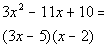*Factor by trial and error

 There is no more factoring that we can do in this problem. Note that if we would multiply this out, we would get the original polynomial.Answer/Discussion to 1cThe first thing that we always check when we are factoring is WHAT? The GCF.  In this case, there is not one.  So we assess what we have. This is a polynomial with four terms.  Looks like we will have to try factoring it by grouping: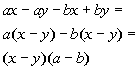*Group in two's *Factor out the GCF out of each separate (   ) *Factor out the GCF of (x  - y)

 There is no more factoring that we can do in this problem. Note that if we would multiply this out, we would get the original polynomial.Answer/Discussion to 1d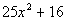Since this is a SUM of two squares it DOES NOT factor. That means this polynomial is prime.Answer/Discussion to 1e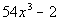The first thing that we always check when we are factoring is WHAT? The GCF.  In this case, there is one.  Factoring out the GCF of x we get: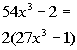*Factor a 2 out of every term

 Next, we assess to see if there is anything else that we can factor. This fits the form of the difference of two cubes.  So we will factor using that rule: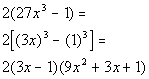*Fits the form of a diff. of two cubes *Binomial is diff. of bases *Trinomial is 1st base squared, plus prod. of bases, plus 2nd base squared

 There is no more factoring that we can do in this problem. Note that if we would multiply this out, we would get the original polynomial.Answer/Discussion to 1f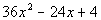The first thing that we always check when we are factoring is WHAT? The GCF.  In this case, there is one.  Factoring out the GCF of x we get: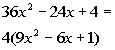*Factor a 4 out of every term

 Next, we assess to see if there is anything else that we can factor. This fits the form of the perfect square trinomial.  So we will factor using that rule: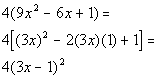*Fits the form of a perfect sq. trinomial *Factor as the diff. of bases squared

 There is no more factoring that we can do in this problem. Note that if we would multiply this out, we would get the original polynomial.

Last revised on Dec. 13, 2009 by Kim Seward.
All contents copyright (C) 2002 - 2010, WTAMU and Kim Seward. All rights reserved.